# Getting to Know grid Graphics

Paul Murrell, The University of Auckland, June 2015

An overview of the short course

# Introduction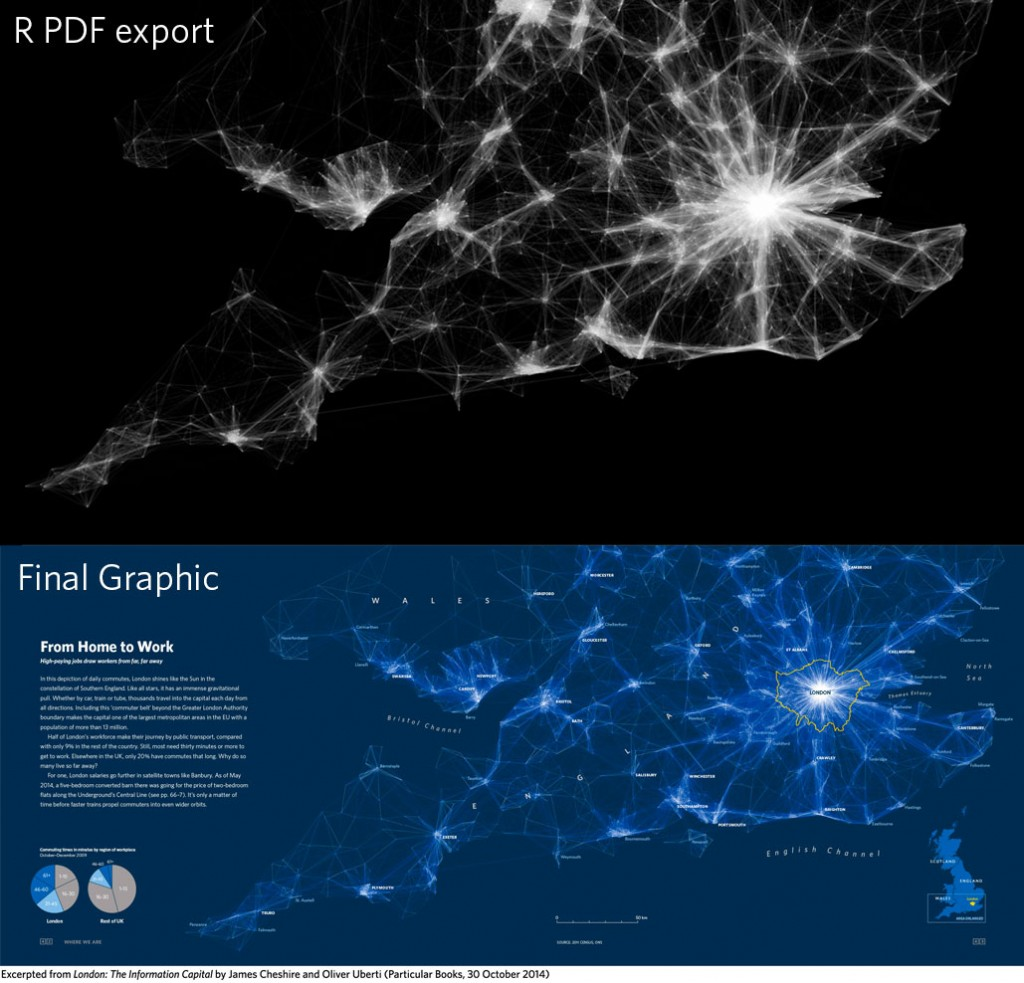For the book "London: The Information Capital", each infographic was begun in R and then "finished off" in Adobe Illustrator. This sort of thing bugs me because I have a deep need to do all of my drawing in code (for keeping a record and for replication and for sharing, among other things).

# Introduction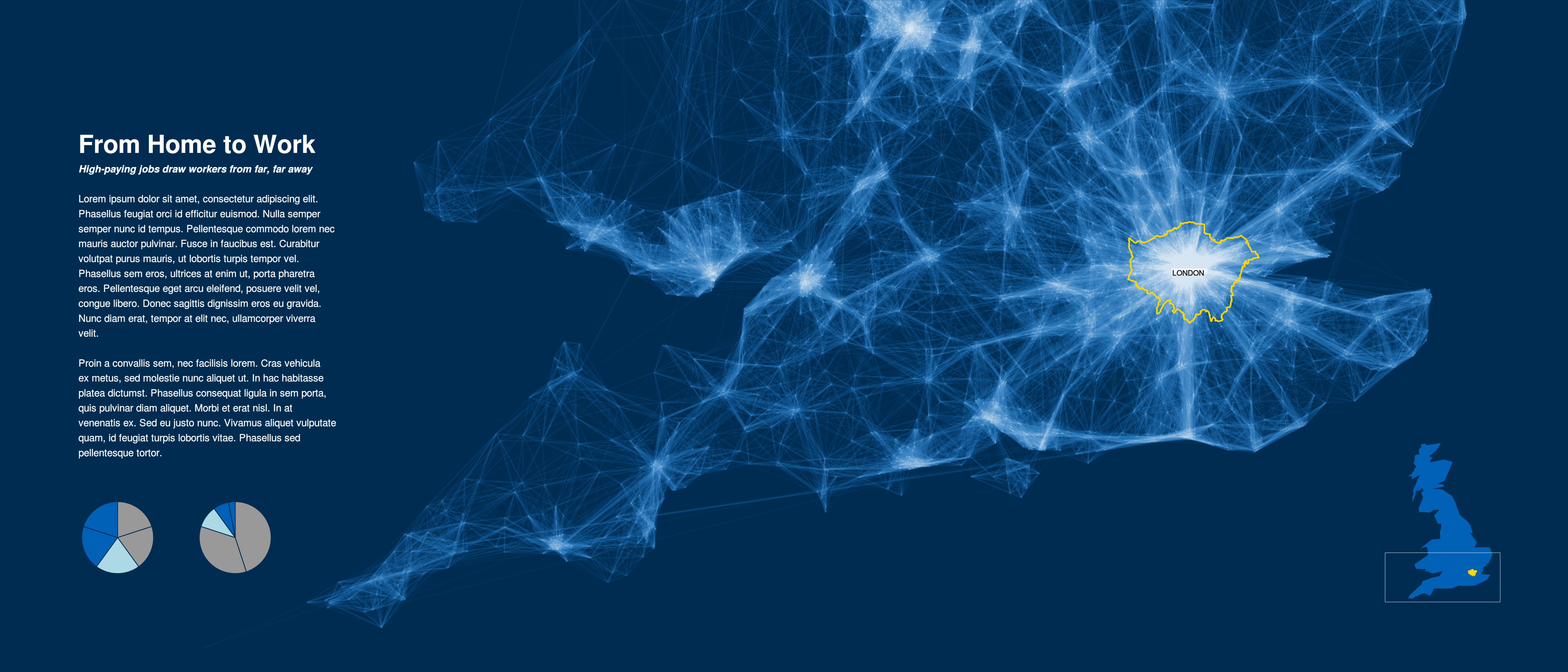One of the distinguishing features of the R graphics system, and the 'grid' graphics system in particular, is that it allows you fine control over details, including access to more advanced graphical features and details. As a "dramatic" demonstration of this idea, the image on this slide was generated completely in R. This course will try to reveal how 'grid' works so that you can do this sort of thing yourself.

# Where is grid ?The 'grid' package provides an ALTERNATIVE graphics system to the 'graphics' package ("base" graphics). Many packages define plotting functions based on 'graphics', but there are some important ones based on 'grid', such as 'lattice' and 'ggplot2'.

# Where is grid ?

```  library(lattice)
xyplot(mpg ~ disp, mtcars)
```When you draw a plot with 'lattice' or 'ggplot2', the actual drawing is being done by 'grid'.

# Exploring grid Grobs

```  library(grid)
grid.ls()
```
```  plot_01.background
plot_01.xlab
plot_01.ylab
plot_01.ticks.top.panel.1.1
plot_01.ticks.left.panel.1.1
plot_01.ticklabels.left.panel.1.1
plot_01.ticks.bottom.panel.1.1
plot_01.ticklabels.bottom.panel.1.1
plot_01.ticks.right.panel.1.1
plot_01.xyplot.points.panel.1.1
plot_01.border.panel.1.1
```

When you draw something with 'grid', a record is kept of the objects that are drawn. 'grid' calls these objects "grobs" (graphical objects). The grid.ls() function can be used to list the grobs on the current page.

# Exploring grid Grobs

Some other functions that help with exploring grobs:

 `grid.grep(path)` Search for a grob that matches 'path'. `showGrob(gPath)` Highlight grob that matches 'gPath'. `grobBrowser()` SVG version with grob names as tooltips (from the 'gridDebug' package).

# grid Viewports

```  xyplot(mpg ~ disp, mtcars)
```When you draw something with 'grid', a record is also kept of any "viewports" that were created. A viewport is a rectangular sub-region on the page.

# Exploring grid Viewports

```current.vpTree()
```
```  viewport[ROOT]
viewport[plot_01.toplevel.vp]
viewport[plot_01.xlab.vp]
viewport[plot_01.panel.1.1.vp]
viewport[plot_01.panel.1.1.off.vp]
viewport[plot_01.strip.left.1.1.off.vp]
viewport[plot_01.ylab.vp]
viewport[plot_01.figure.vp]
viewport[plot_01.]
viewport[plot_01.strip.1.1.off.vp]
```

The current.vpTree() function can be used to list the viewports on the current page. Unfortunately, the output is pretty messy, but hopefully I have shared with you a little function called formatVPTree() that tidies the output up (the tidied output is shown above).

# Exploring grid Viewports

```  grid.ls(viewports=TRUE, grobs=FALSE)
```
```  ROOT
plot_01.toplevel.vp
plot_01.xlab.vp
plot_01.ylab.vp
plot_01.figure.vp
plot_01.panel.1.1.vp
plot_01.strip.1.1.off.vp
plot_01.strip.left.1.1.off.vp
plot_01.panel.1.1.off.vp
```

The grid.ls() function can also be used to list the viewports on the current page. (The output on this slide has been trimmed and tidied to fit on one slide.)

# Exploring grid Viewports

```  grid.ls(viewports=TRUE, fullNames=TRUE)
```
```  viewport[ROOT]
rect[plot_01.background]
viewport[plot_01.toplevel.vp]
viewport[plot_01.xlab.vp]
text[plot_01.xlab]
upViewport
viewport[plot_01.ylab.vp]
text[plot_01.ylab]
upViewport
viewport[plot_01.figure.vp]
```

This is the first few lines of the complete output from grid.ls() that shows both viewports and grobs (and therefore the nesting of grobs within viewports).

# Exploring grid Viewports

Some other functions that help with exploring viewports:

 `showViewport(vp)` Highlight viewport that matches 'vp'. `current.viewport()` Returns the current viewport.

Ideally, a package will document the naming scheme that it uses for grobs and viewports. Ideally, a package will have a naming scheme!

# Exercise

The purpose of this exercise is to make use of the grid.ls() function.

The following code creates a 'lattice' scatterplot:

```library(lattice)
xyplot(mpg ~ disp, mtcars, main="Fast Cars")
```
1. What is the name of the grob that represents the main title on the scatterplot ?
2. What is the name of the viewport that the main title is drawn within ?

# Why Grobs ?

```library(lattice)
barchart(Party ~ Amount_Donated, sortedTotals)
```One benefit of having access to the low-level 'grid' grobs is that we can make detailed customisations to a plot that was drawn with a high-level function where the high-level function does not provide control over enough of the details. In this case, I want to remove the border around the lattice panel.

# Why Grobs ?

```library(grid)
grid.ls()
```
```  plot_01.background
plot_01.xlab
plot_01.ticks.top.panel.1.1
plot_01.ticklabels.left.panel.1.1
plot_01.ticks.bottom.panel.1.1
plot_01.ticklabels.bottom.panel.1.1
plot_01.abline.v.panel.1.1
plot_01.barchart.abline.v.panel.1.1
plot_01.barchart.rect.panel.1.1
plot_01.border.panel.1.1
```

If I can find out what the grob is called ...

# Why Grobs ?

```library(grid)
grid.remove("plot_01.border.panel.1.1")
```... then I can remove it with grid.remove().

# Working With Grobs

Functions that can be used to access grobs:

 `grid.remove()` Remove a grob. `grid.edit()` Modify a grob component. `grid.get()` Get a copy of a grob component. `grid.set()` Replace a grob component.

Each function takes the name of a grob as its first argument. The name argument can be a regular expression, if you specify 'grep=TRUE'. You can work with more than one grob at once if you specify 'global=TRUE'.

# Modifying Grobs

```library(grid)
t <- grid.get("plot_01.ticklabels.bottom.panel.1.1")
names(t)
```
```    "label"         "x"             "y"             "just"
 "hjust"         "vjust"         "rot"           "check.overlap"
 "name"          "gp"            "vp"
```
```t\$just
```
```   "centre" "top"
```

Grobs are just lists with components.

# Modifying Grobs

```library(grid)
grid.edit("plot_01.ticklabels.bottom.panel.1.1",
just=c("left", "top"))
```We can use grid.edit() to change the value of a component.

However, we may NOT edit 'name' or 'vp' components of a grob.

# Modifying Grobs

```library(grid)
gpar(col="blue", lwd=3, lty="dashed")
```
```  \$col
 "blue"

\$lwd
 3

\$lty
 "dashed"
```

The value of the 'gp' component of a grob is created with the gpar() function.

# Modifying Grobs

Common gpar() settings:

 `col` (border) colour. `fill` fill colour. `lty` line type. `lwd` line width. `cex` text size multiplier.

The other gpar() settings:

 `fontsize` The size of text (in points). `lineheight` Vertical height of a line of text (multiplier). For vertical positioning of multi-line text. `fontface` "plain", "bold", "italic", or "bolditalic". `fontfamily` "sans", "serif", "mono", or the name of a font family that makes sense on the current graphics device. `lineend` "round", "square", or "butt". The shape used at the end of lines. `linejoin` "round", "mitre", "bevel". The shape used at line corners. `linemitre` Number used to decide when mitre joins become bevel joins. `lex` Line expansion multiplier (affects line width).

# Modifying Grobs

```library(grid)
grid.edit("plot_01.ticklabels.bottom.panel.1.1",
gp=gpar(col="grey"))
```Modifying the 'gp' component of a grob ONLY changes the gpar() settings that are given new values.

# Exercise

The purpose of this exercise is to make use of the grid.edit() and grid.remove() functions.

The following code creates a 'lattice' scatterplot:

```library(lattice)
xyplot(mpg ~ disp, mtcars, main="Fast Cars")
```
1. Change the colour of the main title to red.
2. Remove the main title from the plot.

# Why Viewports ?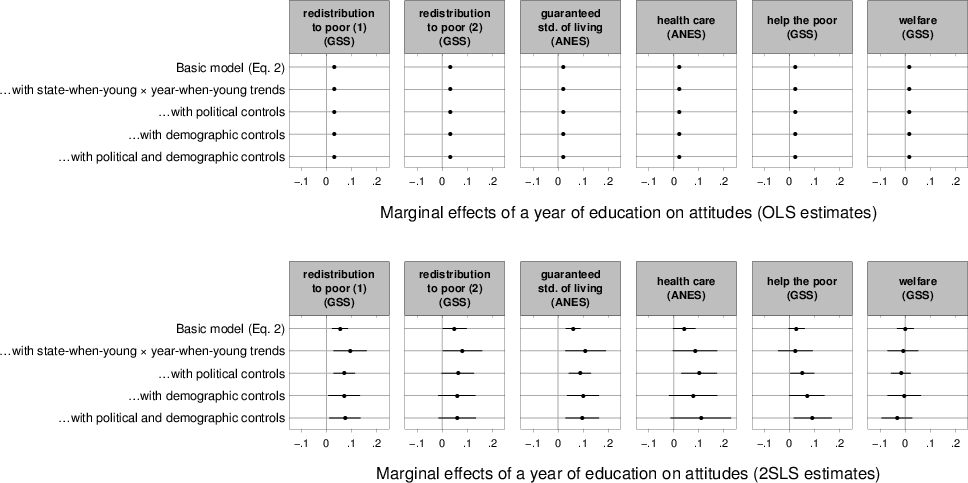Credit: John G. Bullock, Yale University.

John Bullock made use of the 'lattice' viewports that were created in this multi-panel plot to centre the text below each row of plots. (He did not use the 'xlab' argument to xyplot() because he wanted greater control over the vertical placement of the text.)

# Why Viewports ?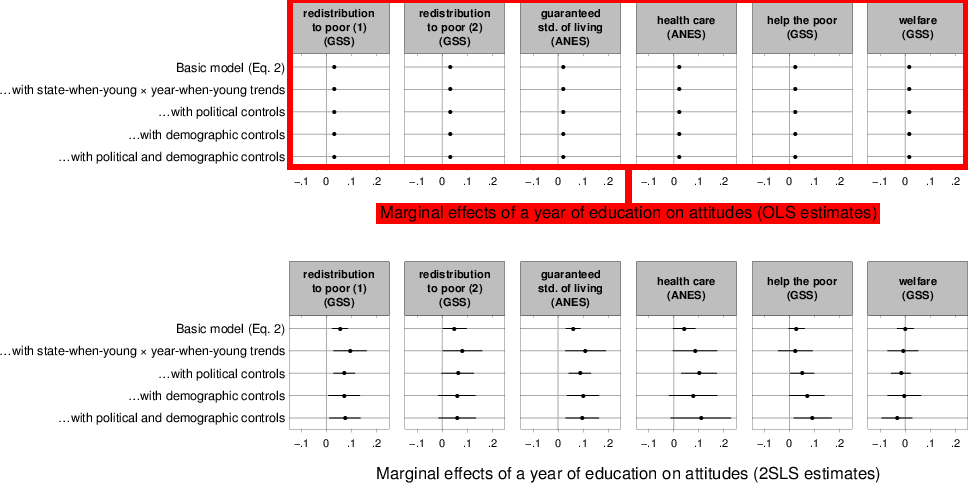Credit: John G. Bullock, Yale University.

John could do this because it is possible to revisit the viewports that are created when a plot is drawn with 'grid'.

# Navigating Viewports

```xyplot(mpg ~ disp, mtcars)
```When you draw something with 'grid', a record is kept of any "viewports" that were created. A viewport is a rectangular sub-region on the page.

# Navigating Viewports

```current.vpTree()
```
```  viewport[ROOT]
viewport[plot_01.toplevel.vp]
viewport[plot_01.xlab.vp]
viewport[plot_01.panel.1.1.vp]
viewport[plot_01.panel.1.1.off.vp]
viewport[plot_01.strip.left.1.1.off.vp]
viewport[plot_01.ylab.vp]
viewport[plot_01.figure.vp]
viewport[plot_01.]
viewport[plot_01.strip.1.1.off.vp]
```

Viewports can be nested within each other.

# Navigating ViewportsViewports can be nested within each other.

# Navigating Viewports

```downViewport("plot_01.toplevel.vp")
grid.rect(gp=gpar(col=NA, fill=rgb(0,1,0,.5)))
```It is possible to revisit the viewports that a plot has created with the downViewport() function (and the name of the viewport we want to visit).

# Navigating Viewports

```downViewport("plot_01.panel.1.1.vp")
grid.rect(gp=gpar(col=NA, fill=rgb(1,0,0,.5)))
```It is possible to revisit the viewports that a plot has created with the downViewport() function (and the name of the viewport we want to visit).

NOTE that drawing occurs relative to the viewport that we are currently in (by default, grid.rect() fills the entire viewport).

# Navigating Viewports

```upViewport()
grid.rect(gp=gpar(col=NA, fill=rgb(0,0,1,.5)))
```We can navigate up the viewport tree as well, using the upViewport() function.

# Exercise

The purpose of this exercise is to make use of the downViewport() function.

The following code creates a 'lattice' scatterplot:

```library(lattice)
xyplot(mpg ~ disp, mtcars, main="Fast Cars")
```
1. Navigate to the viewport that the main title was drawn in and draw a rectangle to show that you are in the right place.

# Exercise

This is the result you are looking for:# Why Viewports ?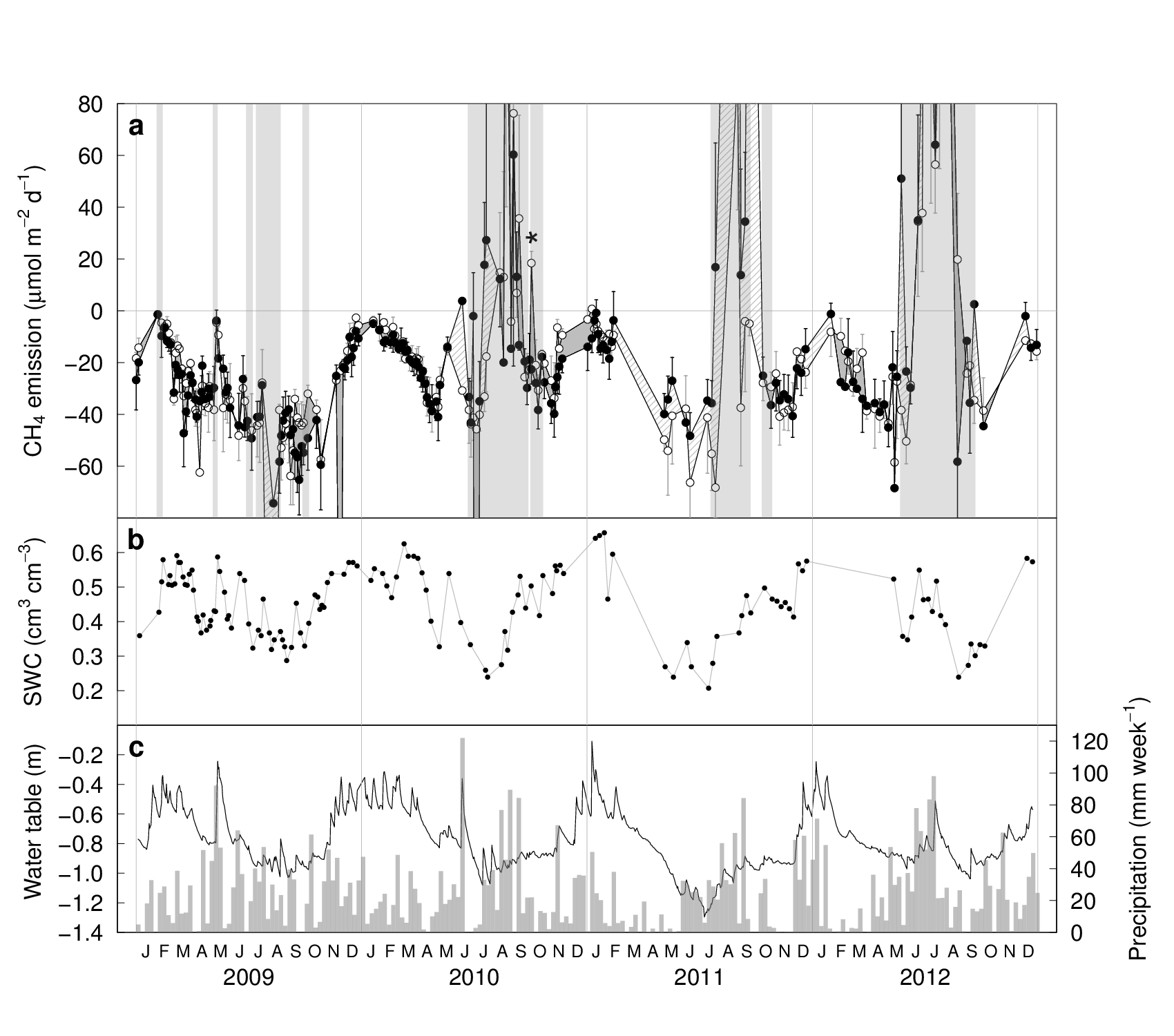Credit: Pascal A. Niklaus, University of Zurich.

Pascal Niklaus wanted to add letter labels in the top-left corner of each of his plots.

# Why Viewports ?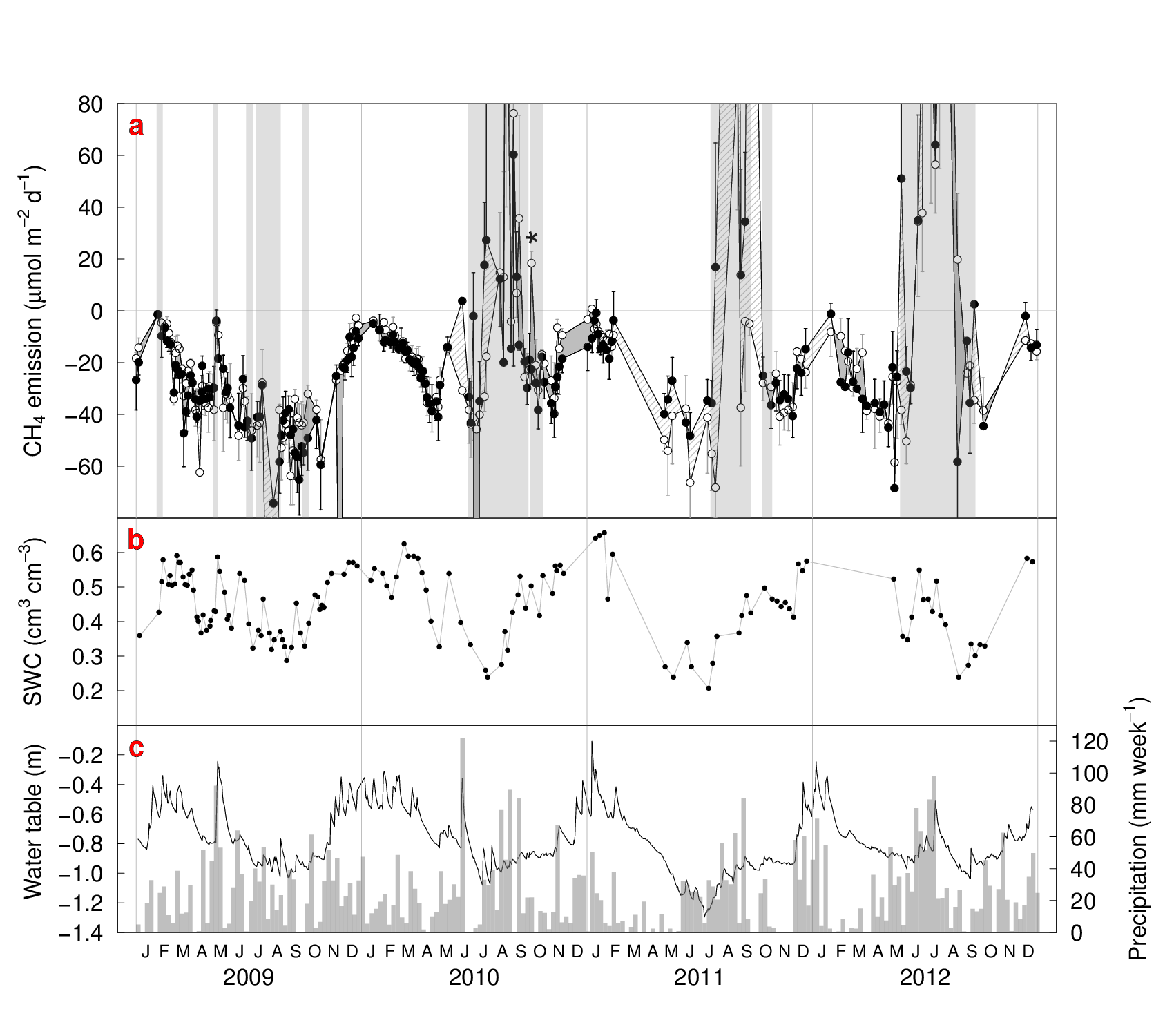Credit: Pascal A. Niklaus, University of Zurich.

These letter labels are difficult to position using the coordinate system provided by the scales on the plot axes.

# Why Viewports ?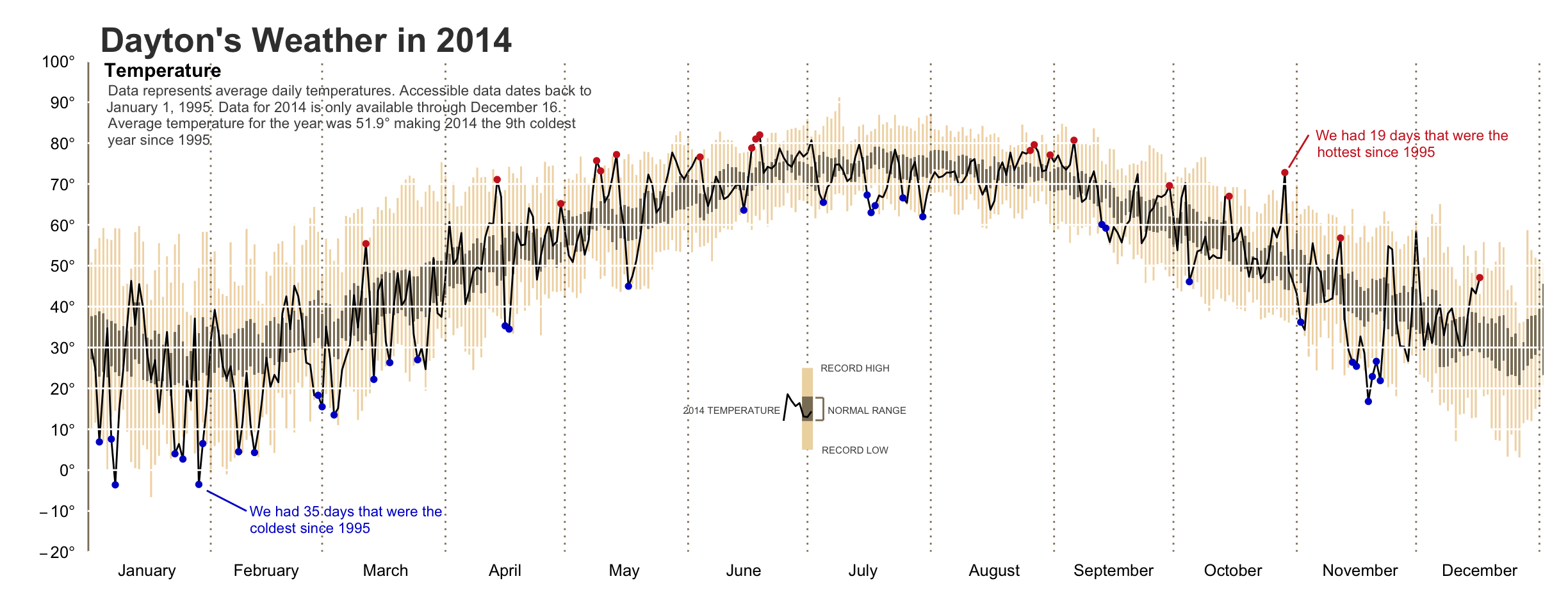Here is a similar example, with more elaborate text annotations, this time on a 'ggplot2' plot.

# Viewport Coordinates

```xyplot(mpg ~ disp, mtcars)
downViewport("plot_01.panel.1.1.vp")
```Every 'grid' viewport has several coordinate systems associated with it.

# Viewport Coordinates

```grid.text("native",
x=unit(300, "native"), y=unit(30, "native"),
just=c("left", "bottom"))
```The unit() function associates a value with a coordinate system.

"Native" coordinates are relative to the 'xscale' and 'yscale' on the viewport.

# Viewport Coordinates

```grid.text("absolute",
x=unit(1, "in"), y=unit(1, "cm"),
just=c("left", "bottom"))
```Absolute coordinates include "in", "cm", and "pt". All are measured from the bottom-left corner of the viewport.

# Viewport Coordinates

```grid.text("normalised",
x=unit(.75, "npc"), y=unit(.5, "npc"),
just=c("left", "bottom"))
```Normalised ("npc") coordinates are 0-to-1 along each side of the viewport.

# Viewport Coordinates

```grid.text("normalised - absolute",
x=unit(1, "npc") - unit(1, "cm"),
y=unit(1, "npc") - unit(1, "cm"),
just=c("right", "top"))
```We can use simple arithmetic on units. In this case, we are positioning text a fixed amount in from the top-right corner of a viewport.

# Drawing Grobs

Some basic shapes that 'grid' can draw:

 `grid.rect(x,y,w,h)` rectangles. `grid.circle(x,y,r)` circles. `grid.lines(x,y)` straight lines through (x,y). `grid.segments(x0,y0,x1,y1)` straight lines from (x0,y0) to (x1,y1). `grid.text(label,x,y)` text.

The other "shapes" that 'grid' can draw:

 `grid.move.to(x,y)` set current "pen" location. `grid.line.to(x,y)` straight line from "pen" location to (x,y) (and set "pen" location to (x,y)). `grid.polyline(x,y,id)` straight lines through multiple sets of (x,y). `grid.xspline(x,y,shape)` smooth curve relative to (x,y) control points. `grid.roundrect(x,y,w,h,r)` rectangle with rounded corners. `grid.polygon(x,y)` polygon defined by (x,y) (automatically closing last point to first point). `grid.path(x,y,id)` path (potentially defined by multiple polygons). `grid.raster(image,x,y,w,h)` raster image. `grid.curve(x1,y1,x2,y2)` smooth curve between two end points. `grid.points(x,y,pch)` data symbols at (x,y) (default coordinate system is "native"!).

# Drawing Grobs

```grid.rect(x=unit(.2, "npc"), y=unit(100, "native"),
width=unit(1, "in"), height=unit(1, "lines"))
```Whenever we draw a grob, we can specify the position and size of the grob using any of the available coordinate systems (the default is almost always "npc").

# Drawing Grobs

```grid.circle(x=1:5/6, y=.5, r=1:5/25)
```For most grobs, the location and size specifications can be vectors so that a single call to a 'grid' function can produce multiple shapes.

# Exercise

The purpose of this exercise is to make use of the unit() function as well as 'grid' functions that draw basic shapes.

The following code creates a 'lattice' scatterplot:

```library(lattice)
xyplot(mpg ~ disp, mtcars, main="Fast Cars")
```
1. Navigate to the viewport that the data symbols were drawn in and draw a horizontal line at mpg == 25.
2. Draw a label just above and to the right of the symbol for the "Pontiac Firebird" (disp=400, mpg=19.2).

# Exercise

This is the result you are looking for:# Why Viewports ?Credit: Tom Wright, affiliation unknown.

Tom Wright has a 'lattice' plot with too many empty panels.

# Why Viewports ?We can do a little bit better if we draw separate 'lattice' plots in separate 'grid' viewports.

# Why Viewports ?This version of the "improved" plot has the 'grid' viewports highlighted by coloured rectangles.

# Starting a New Page

```grid.newpage()
```With raw 'grid', we have to explicitly call the grid.newpage() function to start a new page/screen. All other 'grid' functions just add to the current page/screen.

'lattice' plotting functions, like xyplot(), automatically start a new page, unless you tell them not to with something like print(xyplot(...), newpage=FALSE).

# Creating Viewports

```vp <- viewport(width=.5, height=.5)
pushViewport(vp)
grid.rect(gp=gpar(col=NA, fill="grey80"))
```We can create a new viewport description with the viewport() function. In this case, we are describing a viewport that is half the width of the page and half the height of the page (the default is to be centred on the middle of the page). The location and size of viewports are specified using the unit() function, just like for grobs (and the default coordinate system is "npc").

We create a viewport on the page using the pushViewport() function. After the "push", all drawing occurs within that viewport. In this case, we draw a rectangle that shows the extent of the viewport that we have created.

# Creating Viewports

```vp2 <- viewport(x=0, y=.5, width=.5, height=.5,
just=c("left", "bottom"))
pushViewport(vp2)
grid.rect()
```When we push a viewport, the viewport description is always relative to the current viewport. In this case, we define and push a viewport that is in the top-left quarter of the current viewport. We draw a rectangle to show the extent of this new viewport.

# Creating Viewports

```grid.newpage()
pushViewport(vp)
print(xyplot(mpg ~ disp, mtcars), newpage=FALSE)
```A 'lattice' plot is just a lot of pushing viewports and drawing shapes.

We can tell a 'lattice' plot to do its pushing of viewports and drawing of shapes in the current viewport rather than starting a new page all for itself. We do this by explicitly calling print() on the plot and setting the 'newpage' argument to FALSE.

# Exercise

The purpose of this exercise is to make use of the viewport() and pushViewport() functions.

The following code creates a 'lattice' scatterplot:

```library(lattice)
xyplot(mpg ~ disp, mtcars, main="Fast Cars")
```
1. Create a viewport in the bottom-right quarter of the page and draw the plot in that viewport.

# Exercise

This is the result you are looking for:# Summary

• 'lattice' plots create 'grid' grobs and viewports.
• grid.ls() lists grobs and viewports.
• grid.remove() and grid.edit() modify grobs.
• downViewport() and upViewport() navigate between viewports.
• grid.rect(), grid.text(), etc draw new grobs.
• gpar() controls graphical parameters.
• viewport() and pushViewport() create new viewports.
• 'lattice' plots can be drawn within any viewport.

Nuff said

# Where is grid ?

```library(ggplot2)
qplot(disp, mpg, data=mtcars, main="Fast Cars")
```When you draw a plot with 'lattice' or 'ggplot2', the actual drawing is being done by 'grid'.

# Exploring grid Grobs

```grid.ls()
```
```  GRID.gTableParent.505
background.1-5-6-1
spacer.4-3-4-3
panel.3-4-3-4
grill.gTree.483
panel.background.rect.474
panel.grid.minor.y.polyline.476
panel.grid.minor.x.polyline.478
panel.grid.major.y.polyline.480
panel.grid.major.x.polyline.482
geom_point.points.470
```

One difference between the grobs created by 'lattice' and the grobs created by 'ggplot2' is that the latter are arranged in a hierarchy. For example, there is a "panel" grob, with a "grill" as its child, and various rectangles and polylines as children of that.

Another difference is that the 'ggplot2' naming scheme is less intuitive and less complete (and undocumented).

# Modifying Grobs

```grid.edit("title.2-4-2-4", gp=gpar(col="red"))
```We can use grid.edit() to change the value of a component just like with 'lattice' plots.

# Modifying Grobs

```grid.remove("title.2-4-2-4")
```We can use grid.remove() to remove grobs just like with 'lattice' plots.

# Modifying Grobs

```library(devtools)
# Install Paul's fork of 'gtable'
install_github("pmur002/gtable")
library(ggplot2)
# Draw plot
qplot(disp, mpg, data=mtcars, main="Fast Cars")
# SINGLE grob!
grid.ls()
# "Force" drawing of 'gtable' grobs
grid.force()
# MANY grobs!
grid.ls()
```

Normally, grid.edit() and grid.remove() CANNOT see ALL 'ggplot2' grobs.

The code above provides a recipe for exposing all 'ggplot2' grobs to grid.edit() and grid.remove().

# Navigating Viewports

```qplot(disp, mpg, data=mtcars, main="Fast Cars")
current.vpTree()
```
```  viewport[ROOT]
viewport[layout]
viewport[spacer.4-3-4-3]
viewport[background.1-5-6-1]
viewport[title.2-4-2-4]
viewport[axis-b.4-4-4-4]
viewport[GRID.VP.86]
viewport[axis]
viewport[axis.1-1-1-1]
viewport[axis.2-1-2-1]
viewport[axis-l.3-3-3-3]
viewport[GRID.VP.87]
viewport[axis]
viewport[axis.1-1-1-1]
viewport[axis.1-2-1-2]
viewport[xlab.5-4-5-4]
viewport[ylab.3-2-3-2]
viewport[panel.3-4-3-4]
```

'ggplot2' creates viewports just like 'lattice'.

# Navigating Viewports

```downViewport("panel.3-4-3-4")
grid.rect(gp=gpar(col=NA, fill=rgb(0,1,0,.5)))
```It is possible to revisit the viewports that 'ggplot2' has created, just like with 'lattice'.

However, one difference is that the viewport representing the 'ggplot2' plot region does NOT have a "native" scale corresponding to the axis scales.

# Exercise

The purpose of this exercise is to make use of 'grid' functions with a 'ggplot2' plot.

The following code creates a 'ggplot2' scatterplot:

```library(ggplot2)
qplot(disp, mpg, data=mtcars, main="Fast Cars")
```
1. Navigate to the viewport that the main title was drawn in and draw a rectangle to show the extent of the viewport.
2. Edit the title grob to move it to the right-hand edge of the plot.
3. Remove the rectangle that you just drew.

# Exercise

This is the result you are looking for:# Where is grid ?

```library(vcd)
mosaic(Titanic)
```A number of other packages build on top of 'grid' (or 'lattice' or 'ggplot2'), but there is no guarantee of a naming scheme for grobs and viewports.

# Exploring grid Grobs

```grid.ls()
```
```  GRID.lines.752
GRID.lines.753
disc:Class=1st,Sex=Male,Age=Child,Survived=No,
circle:Class=1st,Sex=Male,Age=Child,Survived=No,
rect:Class=1st,Sex=Male,Age=Child,Survived=Yes
GRID.lines.754
GRID.lines.755
disc:Class=1st,Sex=Female,Age=Child,Survived=No,
```

# Where is grid ?The 'gridGraphics' package provides a way to convert plots drawn with the 'graphics' package into identical plots drawn with 'grid'.

# Where is grid ?

```plot(mpg ~ disp, mtcars, pch=16, main="Fast Cars")
library(gridGraphics)
grid.echo()
```We can draw a plot based on 'graphics' then call grid.echo() to convert it into 'grid' grobs and viewports.

# Exploring grid Grobs

```grid.ls()
```
```  graphics-background
graphics-plot-1-points-1
graphics-plot-1-bottom-axis-line-1
graphics-plot-1-bottom-axis-ticks-1
graphics-plot-1-bottom-axis-labels-1
graphics-plot-1-left-axis-line-1
graphics-plot-1-left-axis-ticks-1
graphics-plot-1-left-axis-labels-1
graphics-plot-1-box-1
graphics-plot-1-main-1
graphics-plot-1-xlab-1
graphics-plot-1-ylab-1
```

# Navigating Viewports

```current.vpTree()
```
```  viewport[ROOT]
viewport[graphics-root]
viewport[graphics-inner]
viewport[graphics-figure-1-clip]
viewport[graphics-plot-1]
viewport[graphics-window-1-0]
viewport[graphics-window-1-1]
viewport[graphics-figure-1]
viewport[graphics-plot-1]
viewport[graphics-window-1-0]
viewport[graphics-window-1-1]
viewport[graphics-plot-1-clip]
viewport[graphics-window-1-0]
viewport[graphics-window-1-1]
```

# Exercise

The purpose of this exercise is to make use of 'grid' functions with a 'graphics' plot.

The following code creates a 'graphics' scatterplot:

```plot(mpg ~ disp, mtcars, pch=16, main="Fast Cars")
```
1. Convert the plot to an identical 'grid' plot, change the title to red.
2. Remove the title.

# Exercise

This is the result you are looking for (before you delete the title):# Why grid ?The concepts of viewports in the 'grid' package provide a better basis (than the 'graphics' package) for producing complex plots like 'lattice' multi-panel conditioning plots.

For example, how does 'lattice' leave enough space for the axis labels and the plot legend, but make the panels as large as possible ?

# Why grid ?Plots produced by 'ggplot2' also benefit from 'grid' concepts like viewports to determine the arrangement of pieces of a complex plot within a page.

# Why grid ?The fact that 'grid' is a general-purpose graphics system (not just for plots) means that 'grid' concepts like viewports can be applied to things like arranging photos together on a page.

# Why grid ?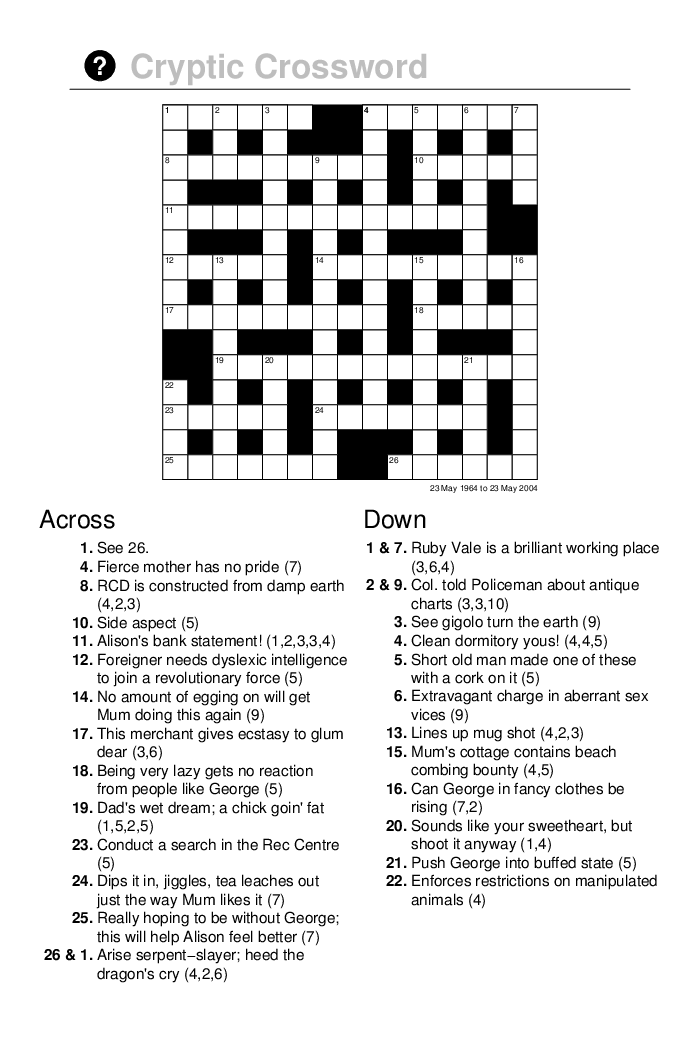The fact that 'grid' is a general-purpose graphics system also means that 'grid' can be used as a rudimentary desktop publishing system (code-based, of course).

# grid LayoutsThe approach that 'lattice' takes is to use a 'grid' "layout". A layout divides up a (parent) viewport into a number of rows and columns and subsequent (child) viewports that are pushed beneath the (parent) viewport with the layout can occupy a subset of the cells in the (parent) layout.

# Creating Layouts

```widths <- unit(c(1, 2, 1), c("null", "null", "cm"))
lay <- grid.layout(3, 3, widths=widths)
vplay <- viewport(layout=lay)
pushViewport(vplay)
pushViewport(viewport(layout.pos.row=2,
layout.pos.col=2))
grid.rect(gp=gpar(col=NA, fill=rgb(1,0,0,.5)))
```A layout can be specified as part of the description of a viewport.

A viewport can specify its location in terms of the rows and columns of a parent layout.

# Exercise

The purpose of this exercise is to make use of the grid.layout() function.

1. Produce a layout like the diagram below.
```       +-----------------------------------------+
|         w=7/9      5    h=0.5/5.5       |
+-----+-----------------------------+-----+
|     |              1    h=1.0/5.5 |     |
|     +-----------------------------+     |
|  6  |              2    h=1.0/5.5 |  7  |
|     +-----------------------------+     |
|w=1/9|              3    h=1.0/5.5 |w=1/9|
|     +-----------------------------+     |
|     |                             |     |
|     |              4    h=2.0/5.5 |     |
|     |                             |     |
+-----+-----------------------------+-----+```

Credit: Julio Sergio Santana.

# Exercise

This is the result you are looking for (the grid.show.layout() function takes a 'grid' layout as its argument and draw a diagram of the layout):# Viewport Coordinates

```grid.rect(width=stringWidth("axis label"))
```The stringWidth() function lets us specify a width in terms of the size of a piece of text (like a label on a plot axis).

There is also a stringHeight() function.

Both of these functions can be used to specify the widths of columns (or heights of rows) in a 'grid' layout (for example to leave enough space for axis labels around a plot).

# Viewport Coordinates

```grid.text("axis label", name="t")
grid.rect(width=grobWidth("t"))
```The grobWidth() function lets us specify a width in terms of the size of a grob (even a collection of grobs like a plot legend).

There is also a grobHeight() function.

Both of these functions can also be used to specify the widths of columns (or heights of rows) in a 'grid' layout (for example to leave enough room for a legend beside a plot).

# Why grid ?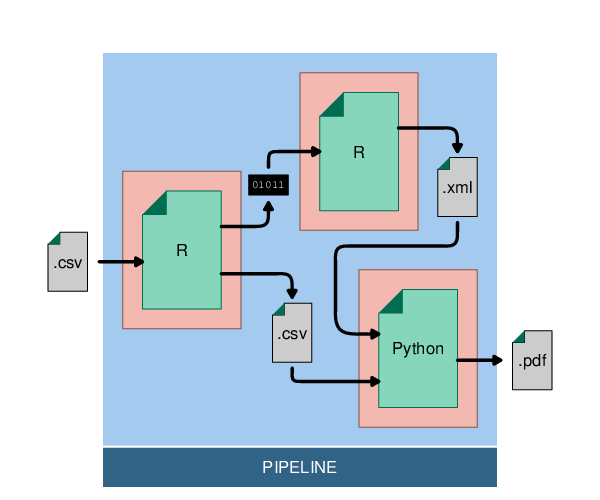This sort of diagram presents a different sort of problem: how to draw a line from the edge of one shape to the edge of another shape ?

# Viewport Coordinates

```grid.text("label", x=1/3, y=1/3, name="t")
grid.circle(2/3, 2/3, r=unit(1, "mm"),
gp=gpar(fill="black"))
grid.segments(grobX("t", 0), grobY("t", 0), 2/3, 2/3)
```The grobX() function lets us specify a location in terms of the boundary of a grob.

There is also a grobY() function.

# Exercise

The purpose of this exercise is to make use of the grobX(), grobY(), grobWidth(), and grobHeight() functions.

The following code draws two pieces of text:

```grid.newpage()
grid.text("label 1", 1/3, 2/3, name="l1")
grid.text("label two", 2/3, 1/3, name="l2")
```
1. Draw a circle around each piece of text (with a radius that is half of the width of the text, plus 1mm).
2. Draw a line from the bottom-right edge of the top-left circle to the top-left edge of the bottom-right circle.

# Exercise

This is the result you are looking for:# Why grid ?This sort of infographic is an example of something that is hard to do in standard R graphics because it involves more advanced graphics operations (like raster image compositing).

# Where is grid ?The 'gridSVG' package provides access to some of the more sophisticated graphics features of SVG.

# gridSVG

```library(gridSVG)
grid.circle(r=.2, name="c")
grid.filter("c", filterEffect(feGaussianBlur(sd=3)))
grid.export()
```The gridsvg() function opens a 'gridSVG' device. An alternative is the grid.export() function.

# Exercise

The purpose of this exercise is to make use of the 'gridSVG' package.

The following code draws a scatterplot:

```library(lattice)
xyplot(jitter(Sepal.Length) ~ jitter(Sepal.Width),
group=Species, iris,
par.settings=list(
superpose.symbol=list(pch=21,
col="black",
fill="grey")))
```
1. Blur all of the points except for the "setosa" variety (hint: there are three separate points grobs in the plot, one for each variety).

# Exercise

This is the result you are looking for
(which is an SVG file):# Summary

• 'ggplot2' plots create 'grid' grobs and viewports.
• Other packages create 'grid' grobs and viewports too, but there is no guarantee of a naming scheme.
• 'gridGraphics' creates 'grid' grobs and viewports from 'graphics' plots.
• grid.layout() creates layouts.
• grobX(), grobY(), grobWidth(), and grobHeight() describe locations and sizes in terms of the locations and sizes of other grobs.
• 'gridSVG' exports 'grid' grobs and viewports to SVG, with the possibility of fancier graphics features.

Nuff said

# Writing for Others

• Name all grobs and viewports.